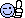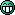# How to translate this Erlang code to LFE?

Hello everyone,

I have been trying to solve AOC with LFE, yet there is something I don’t understand.

This is the Erlang code…

``````-module(day6).

List = binary:split(string:trim(Bin), <<",">>, [global, trim]),
lists:map(fun(X) ->
{V, _} = string:to_integer(X),
V
end, List).
``````

and this almost the same in LFE

``````(defmodule day6 (export (load_data 0)))

(let
(lists:map
(lambda (x) (string:to_integer x))
(string:split (string:trim bin) "," 'all))))
``````

I cannot figure how to replace this

``````    fun(X) ->
{V, _} = string:to_integer(X),
V
end
``````

and this would be the LFE code.

``````(lambda (x) (string:to_integer x))
``````

but string:to_integer x returns a tuple, and I am only interested in the first value.

How can I return only the first element?

Thanks for taking time.

2 Likes

Sorry for the question… I just found the solution after posting.

``````(defun load_data ()
(let
(lists:map
(lambda (x) (let (((tuple y _) (string:to_integer x))) y))
(string:split (string:trim bin) "," 'all))))
``````

It is working as expected. I did not fully understood the let macro2 Likes

My lfe skills are not great but can’t you just let bind the value, just as you do for your `file:read_file`?

``````(lambda (x)
(let (((tuple v _) (string:to_integer x)))
v))
``````

Or you could use `element/2`

``````(lambda (x) (element 1 (string:to_integer x)))
``````

EDIT: Oops, late to the party4 Likes

Thank You for your reply, I figure it out also, I have to say it’s my first Lisp code3 Likes

All I can say here is that the solutions are correct.

7 Likes

Congrats Koko!I hope to learn a Lisp one day… and I think LFE would be perfectif we see a PragProg book from Duncan (or Robert) I will add it further up my list2 Likes

Since then I made an AOC problem with LFE.

I have read the books from @oubiwann and followed the start of this course.

Here is the secret I have learned``````# Elixir
input |> ... |> ... |>

%% Erlang
S0=...(Input).
S1=...(S0).
S3=...(S1).

; LFE
(...(...(...input)))
``````
3 Likes

@oubiwann has written a lot of great stuff for/about/using LFE.

3 Likes

``````(-> input